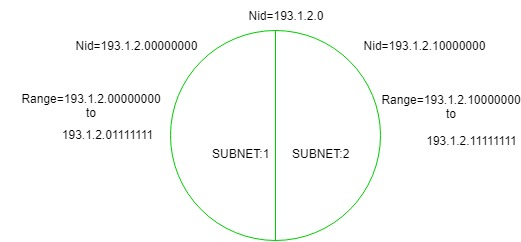# Introduction To Subnetting

• Difficulty Level : Easy
• Last Updated : 07 Jun, 2022

When a bigger network is divided into smaller networks, to maintain security, then that is known as Subnetting. So, maintenance is easier for smaller networks. Now, let’s talk about dividing a network into two parts: To divide a network into two parts, you need to choose one bit for each Subnet from the host ID part.In the above diagram, there are two Subnets. Note: It is a class C IP so, there are 24 bits in the network id part and 8 bits in the host id part.

• For Subnet-1: The first bit which is chosen from the host id part is zero and the range will be from (193.1.2.00000000 till you get all 1’s in the host ID part i.e, 193.1.2.01111111) except for the first bit which is chosen zero for subnet id part. Thus, the range of subnet-1:
`193.1.2.0 to 193.1.2.127 `
• For Subnet-2: The first bit chosen from the host id part is one and the range will be from (193.1.2.100000000 till you get all 1’s in the host ID part i.e, 193.1.2.11111111). Thus, the range of subnet-2:
`193.1.2.128 to 193.1.2.255 `

Note:

1. To divide a network into four (22) parts you need to choose two bits from the host id part for each subnet i.e, (00, 01, 10, 11).
2. To divide a network into eight (23) parts you need to choose three bits from the host id part for each subnet i.e, (000, 001, 010, 011, 100, 101, 110, 111) and so on.

Example1. An organization is assigned a class C network address of 201.35.2.0. It uses a netmask of 255.255.255.192 to divide this into sub-networks. Which of the following is/are valid host IP addresses?

```A. 201.35.2.129
B. 201.35.2.191
C. 201.35.2.255
D. Both (A) and (C)```

Solution:

Converting the last octet of the netmask into the binary form: 255.255.255.11000000

Converting the last octet of option A into the binary form: 201.35.2.10000001

Converting the last octet of option B into the binary form: 201.35.2.10111111

Converting the last octet of option C into the binary form: 201.35.2.11111111

From the above, we see that Option B and C is not a valid host IP address (as they are broadcast address of a subnetwork)

and OPTION A is not a broadcast address and it can be assigned to a host IP.

Example 2. An organization has a class C network address of 201.32.64.0. It uses a subnet mask of 255.255.255.248. Which of the following is NOT a valid broadcast address for any subnetworks?

```A.  201.32.64.135
B.  201.32.64.240
C.  201.32.64.207
D.  201.32.64.231```

Solution:

Converting the last octet of the netmask into the binary form: 255.255.255.11111000

Converting the last octet of option A into the binary form: 201.32.64.10000111

Converting the last octet of option B into the binary form: 201.32.64.11110000

Converting the last octet of option C into the binary form: 201.32.64.11001111

Converting the last octet of option D into the binary form: 201.32.64.11100111

From the above, we can see that, in OPTION A, C, and D all the host bits are 1 and give the valid broadcast address of subnetworks.

and OPTION B the last three bits of the Host address are not 1 therefore it’s not a valid broadcast address.

My Personal Notes arrow_drop_up